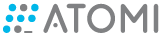# Score subtract value

Problem:

Saola Animate version: 3

OS: w10

Notes:

I’m trying to create a game where there are two scores

Div_red and Div_green

Example:
10 apples on the screen, 4 are green and 6 are red, the target will be the red apples that give points,

div_redshow = 0
div_green show = 0

when I click on the red ones I add (1) score
imagine clicking 4 red I have (4) scores

div_redshow = 4
div_green show = 0

Now I’m going to click on a green one

div_red show = 3
div_green show = 1

Div_red will show the total gain
Div_green will subtract 1 and show the total

I will click on a green one again

div_redshow = 2
div_green show = 2

I tried this code but it didn’t work

function ajust_score(doc, e) {

var a = 1;

doc.score +=1;
doc.getElement(“div_score”).setText(doc.score);
doc.a(a -= doc.score);
doc.getElement(“div_score_1”).setText(doc.a);

}

I appreciate if you can put me on the right paththank you
score.saola (45.4 KB)

Hi,